## Tamilnadu Samacheer Kalvi 6th Maths Solutions Term 1 Chapter 4 Geometry Ex 4.4

Miscellaneous Practice Problems

Question 1.
Find the type of lines marked in thick lines (Parallel, intersecting or perpendicular)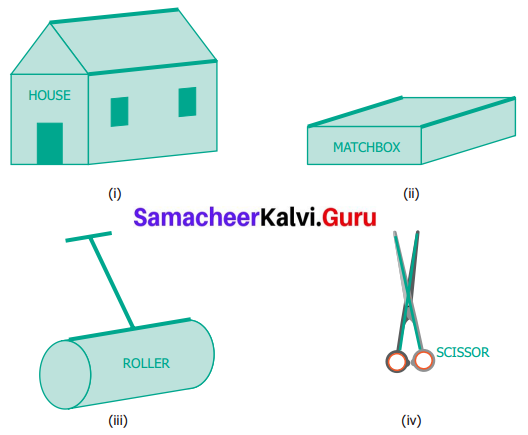Solution:
(i) Parallel lines
(ii) Parallel lines
(iii) Parallel lines and Perpendicular lines
(iv) Intersecting lines

Question 2.
Find the parallel and intersecting line segments in the picture given below.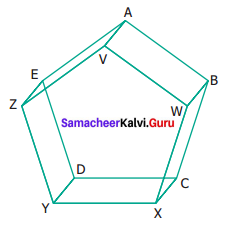Solution:
(a) Parallel line segments

• $$\overline{\mathrm{YZ}} \text { and } \overline{\mathrm{DE}}$$
• $$\overline{\mathrm{EA}} \text { and } \overline{\mathrm{ZV}}$$
• $$\overline{\mathrm{VW}} \text { and } \overline{\mathrm{AB}}$$
• $$\overline{\mathrm{WX}} \text { and } \overline{\mathrm{BC}}$$
• $$\overline{\mathrm{YX}} \text { and } \overline{\mathrm{DC}}$$
• $$\overline{\mathrm{YD}} \text { and } \overline{\mathrm{XC}}$$
• $$\overline{\mathrm{XC}} \text { and } \overline{\mathrm{WB}}$$
• $$\overline{\mathrm{WB}} \text { and } \overline{\mathrm{VA}}$$
• $$\overline{\mathrm{VA}} \text { and } \overline{\mathrm{ZE}}$$
• $$\overline{\mathrm{ZE}} \text { and } \overline{\mathrm{YD}}$$

(b) Intersecting line segments

• DE and ZV
• WX and DC

Question 3.
Name the following angles as shown in the figure.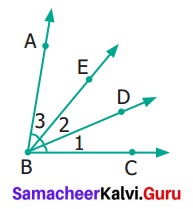Solution:
(i) ∠1 = ∠DBC or ∠CBD
(ii) ∠2 = ∠DBE or ∠EBD
(iii) ∠3 = ∠ABE or ∠EBA
(iv) ∠1 + ∠2 = ∠EBC or ∠CBE
(v) ∠2 + ∠3 = ∠ABD or ∠DHA
(vi) ∠1 + ∠2 + ∠3 = ∠ABC or ∠B or ∠CBAQuestion 4.
Measure the angles of the given figures using a protractor and identify the type of angle as acute, obtuse, right or straight.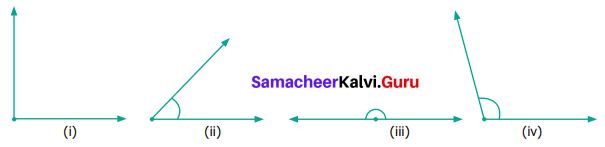Solution:
(i) 90° – Right Angle
(ii) 45° – Acute Angle
(iii) 180° – Straight Angle
(iv) 105° – Obtuse Angle

Question 5.
Draw the following angles using the protractor.
(i) 45°
(ii) 120°
(iii) 65°
(iv) 135°
(v) 0°
(vi) 180°
(vii) 38°
(viii) 90°
Solution:
(i) 45°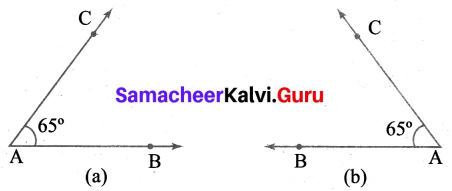Construction:
1. Drawn the base ray PQ.
2. Placed the centre of the protractor at the vertex P. Lined up the ray $$\overrightarrow{\mathrm{PQ}}$$ with the 0° line. Then drawn and labelled a pointed (R) at the 45° mark on the inner scale (a) anticlockwise and (b) outer scale (clockwise)
3. Removed the protractor and drawn at $$\overrightarrow{\mathrm{PR}}$$ to complete the angle
Now ∠P = ∠QPR – ∠RPQ = 45°.

(ii) 120°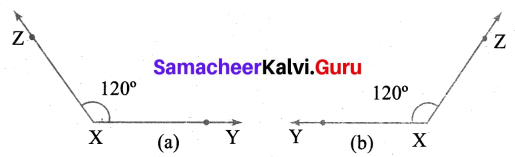Construction:
1. Placed the centre of the protractor at the vertex X. Lined up the ray $$\overline{\mathrm{XY}}$$ with the 0° Line. Then draw and label a point Z at 120° mark on the (a) inner scale (anti-clockwise) and (b) outer scale (clockwise).
2. Removed the protractor and draw $$\overline{\mathrm{XZ}}$$ to complete the angle.
Now, ∠X = ∠ZXY = ∠YXZ = 120°.

(iii) 65°Construction:
1. Placed the centre of the protractor at the vertex A. Line up the ray $$\overrightarrow{\mathrm{AB}}$$ with the 0° line. Then draw and label a point C at the 65° mark on the (a) inner scale (anti-clockwise) (b) outer scale (clockwise).
2. Removed the protractor and draw $$\overrightarrow{\mathrm{AB}}$$ to complete the angle.
Now ∠A = ∠BAC = ∠CAB = 65°.

(iv) 135°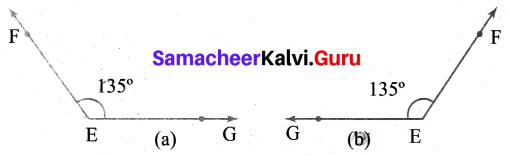Construction:
1. Placed the centre of the protractor at the Vertex A. Lined up the ray $$\overrightarrow{\mathrm{EG}}$$ with the 0° line. Then draw and label a point F at the 135° mark on the (a) inner scale (anti-clockwise) and (b) outer scale (clockwise)
2. Removed the protractor and draw $$\overrightarrow{\mathrm{EF}}$$ to complete the angle.
Now ∠E = ∠FEG = ∠GEF = 135°.

(v) 0°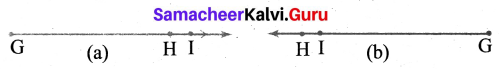Construction:
1. Placed the centre of the protractor at the vertex G. Lined up the ray $$\overrightarrow{\mathrm{GH}}$$ with the 0° line. Then draw and label a point I at the 0° mark on the
(a) inner scale (anti-clockwise)
(b) outer scale (clockwise)
2. Removed the protractor and seen $$\overrightarrow{\mathrm{GI}}$$ lies exactly on $$\overrightarrow{\mathrm{GH}}$$
Now ∠G = ∠HGI = ∠IGH = 0°, which is a zero angle.

(vi) 180°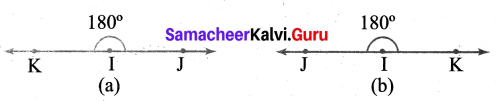Construction:
1. Placed the centre of the protractor at the vertex I. Lined up the ray $$\overrightarrow{\mathrm{IJ}}$$ with the 0° line. Then draw and labelled a point K at the 180° mark on the (a) inner scale (anticlockwise) (b) outer scale (clockwise)
2. Removed the protractor and draw $$\overrightarrow{\mathrm{IK}}$$ to complete the angle.
Now ∠I = ∠JHK = ∠KIJ = 180°, which is a straight Angle.

(vii) 38°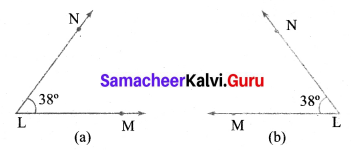Construction:
1. Placed the centre of the protractor at the vertex L. Lined up the ray $$\overrightarrow{\mathrm{LM}}$$ with the 0° line. Then draw and label a point N at 38° mark on the (a) inner scale (anticlockwise) and (b)*huter scale (clockwise).
2. Removed the protractor and draw $$\overrightarrow{\mathrm{LN}}$$ to complete the angle.
Now ∠L = ∠MLN = ∠NLM = 38°.

(viii) 90°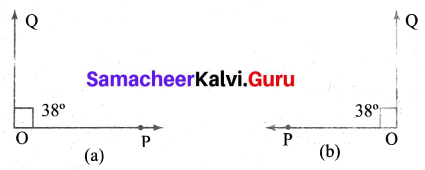Construction:
1. Placed the centre of the protractor at the vertex ‘O’. Lined up the ray $$\overrightarrow{\mathrm{OP}}$$ with the 0° line. Then draw and label a point Q at 90° mark on the (a) inner scale (anticlockwise) and (b) outer scale (clockwise)
2. Removed the protractor and draw $$\overrightarrow{\mathrm{OQ}}$$ to complete the angle.
Now ∠O = ∠POQ = ∠QOP = 90°.Question 6.
From the figures given below, classify the following pairs of angles into complementary and non-complementary.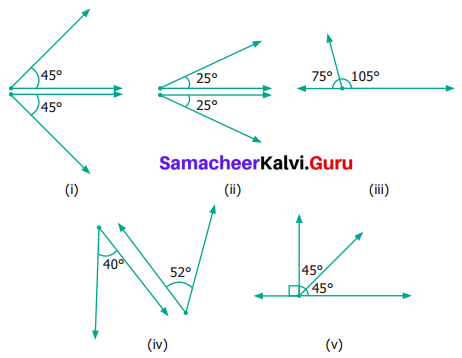Solution:
We know that the two angles are complementary if they add up to 90°.
Therefore (a) (i) is complementary.
In (v) ∠ABC and ∠CBD are complementary
(b) (ii), (iii), (iv) and (v) are non-complementary

Question 7.
From the figures given below, classify the following pairs of angles into supplementary and non-supplementary.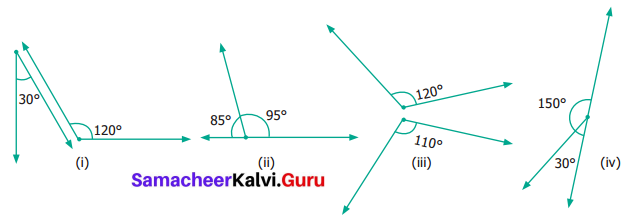Solution:
If two angles add up to 180°, then they are supplementary angles.
(a) In (ii) ∠AOB and ∠BOD are supplementary. In (iv) the pair is supplementary
(b) (i) and (iii) are not supplementary.

Question 8.
From the figure.
(i) name a pair of complementary angles
(ii) name a pair of supplementary angles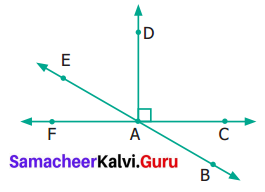Solution:
(i) ∠FAE and ∠DAE are complementary
(ii) ∠FAD and ∠DAC are supplementary

Question 9.
Find the complementary angle of
(i) 30°
(ii) 26°
(iii) 85°
(iv) 0°
Solution:
When we have an angle, how far we need to go to reach the right angle is called the complementary angle.
(i) Complementary angle of 30° is 90° – 30° = 60°
(ii) Complementary angle of 26° is 90° – 26° = 64°
(iii) Complementary angle of 85° is 90° – 85° = 5°
(iv) Complementary angle of 0° is 90° – 0° = 90°
(v) Complementary angle of 90° is 90° – 90° = 0°

Question 10.
Find the supplementary angle of
(i) 70°
(ii) 35°
(iii) 165°
(iv) 90°
(v) 0°
(vi) 180°
(vii) 95°
Solution:
How far we should go in the same direction to reach the straight angle (180°) is called supplementary angle.
(i) Supplementary angle of 70° = 180° – 70° = 110°
(ii) Supplementary angle of 35° is 180° – 35° = 145°
(iii) Supplementary angle of 165° is 180° – 165° = 15°
(iv) Supplementary angle of 90° is 180° – 90° = 90°
(v) Supplementary angle of 0° is 180° – 0° = 180°
(vi) Supplementary angle of 180° is 180° – 180° = 0°
(vii) Supplementary angle of 95° is 180° – 95° = 85°Challenging Problems

Question 11.
Think and write and object having.
(i) Parallel Lines
1. _____________
2. _____________
3. _____________
(ii) Perpendicular lines
1. _____________
2. _____________
3. _____________
(iii) Intersecting lines
1. _____________
2. _____________
3. _____________
Solution:
(i) 1. Opposite edges of a Table.
2. Path traced by the wheels of a car on a straight road
3. Opposite edges of a black board
(ii) 1. Adjacent edges of a Table.
2. Hands of the block when it shows 3.30
3. Strokes of the letter ‘L’
(iii) 1. Sides of a triangle
2. Strokes of letter ‘V’
3. Hands of a scissors

Question 12.
Which angle is equal to twice of its complement?
Solution:
We know that the sum of complementary angles 90°
Given Angle = 2 × Complementary angle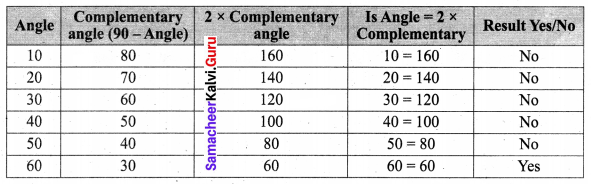By trial and error, we find that Angle = 2 × Complement for 60°
The required angle = 60°
Another method:
Let the angle be x given
x = 2 (90 – x)
⇒ x = 180 – 2x
⇒ x + 2x = 180
⇒ 3x = 180
⇒ x = 60°

Question 13.
Which angle is equal to two-thirds of its supplement.
Solution:
Supplementary angles sum upto 180°
Given Angle = $$\frac{2}{3}$$ × Supplement.
Forming the Table.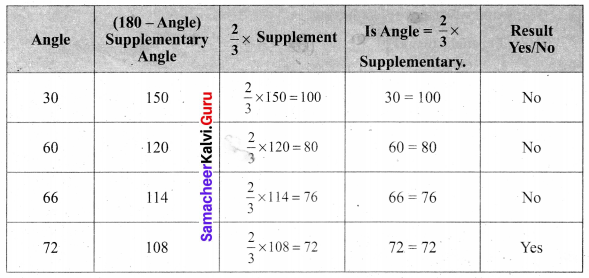By trial and error, we find that angle = $$\frac{2}{3}$$ × supplement for 72°.
The required angle 72°.

Question 14.
Given two angles are supplementary and one angle is 20° more than other. Find the two angles.
Solution:
Given two angles are supplementary i.e. their sum = 180°.
Let the angle be x
Then another angle = x + 20 (given)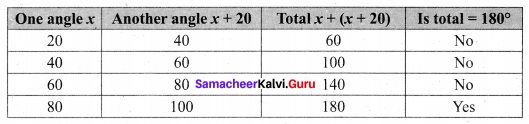The two angles are 80° and 100°.

Question 15.
Two complementary angles are in the ratio 7 : 2. Find the angles.
Solution:
Let the angles be 7x, 2x
According to the problem,
7x + 2x = 90
9x = 90
x = $$\frac{90}{9}$$
x = 10
7x = 7 × 10
= 70
2x = 2 × 10
= 20
∴ Two angles are 70° and 20°Question 16.
Two supplementary angles are in ratio 5 : 4. Find the angles.
Solution:
Total of two supplementary angles = 180°
Given they are in the ratio 5 : 4
Dividing total angles to 5 + 4 = 9 equal parts.
One angle $$=\frac{5}{9} \times 180=100^{\circ}$$
Another angle $$=\frac{4}{9} \times 180=80^{\circ}$$
Two angles are 100° and 80°.